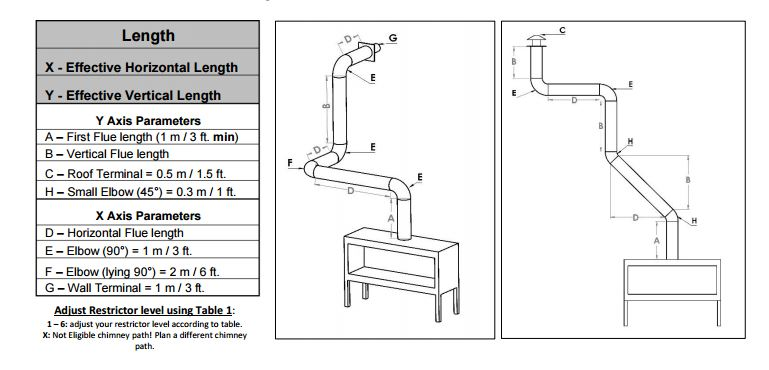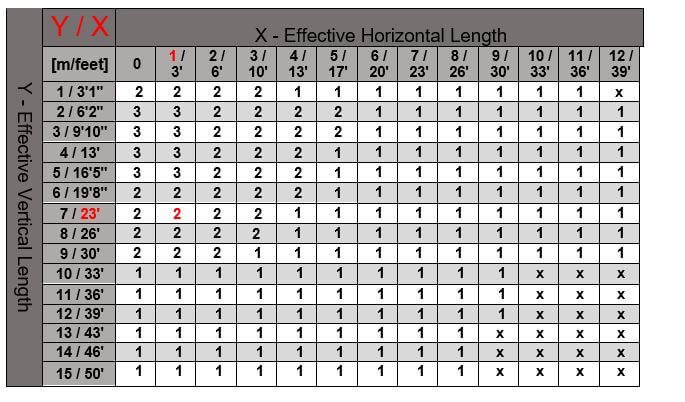### A – First Flue length = 3 = 3 ft B – Vertical Flue length = 1 +15 = 16 ft C – Roof Terminal = 1 = 1.5 ft H – Small Elbow (45°) = 2 = 2 ft

Y Axis Parameters = 22.5 ft### D – Horizontal Flue length = 1 ft E – Elbow (90°) = 0 F – Elbow (lying 90°) = 0 G – Wall Terminal =0

X Axis Parameters = 1